# Game-theoretic Probability

Game-theoretic probability is an alternative approach to the foundations of probability. In some respects it is more flexible than the standard measure-theoretic approach (see measure-theoretic probability) due to Kolmogorov. The game-theoretic framework is as old as the measure-theoretic one, but it was dormant for a long time (see history of game-theoretic probability).

The main connection to on-line prediction is via the technique of defensive forecasting.

From the viewpoint of game theory the framework of game-theoretic probability is very simple. Its basic element is a perfect-information game between Sceptic and World (who is often subdivided into a number of players). Sceptic places bets on events whose outcome is determined by World. A typical result of game-theoretic probability has the following form: Sceptic has a strategy that, when started with the unit initial capital, guarantees that Sceptic's capital is never negative and that either his capital tends to infinity or some interesting property of World's moves is satisfied. As a simple example, see the strong law of large numbers for bounded observations (in which case World is subdivided into two players, Reality and Forecaster).

The sample space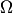is defined to be the set of all possible sequences of moves by World. Suppose all sequences are infinite. Then the upper probability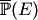for an arbitrary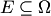is defined as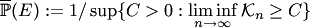where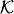ranges over all nonnegative capital processes starting from 1. The definition of upper probability will not change if liminf is replaced by sup (and, therefore, by limsup). A similar definition of upper probability is used in the literature on imprecise probabilities.

Active subareas of game-theoretic probability are:

Here is an open problem: